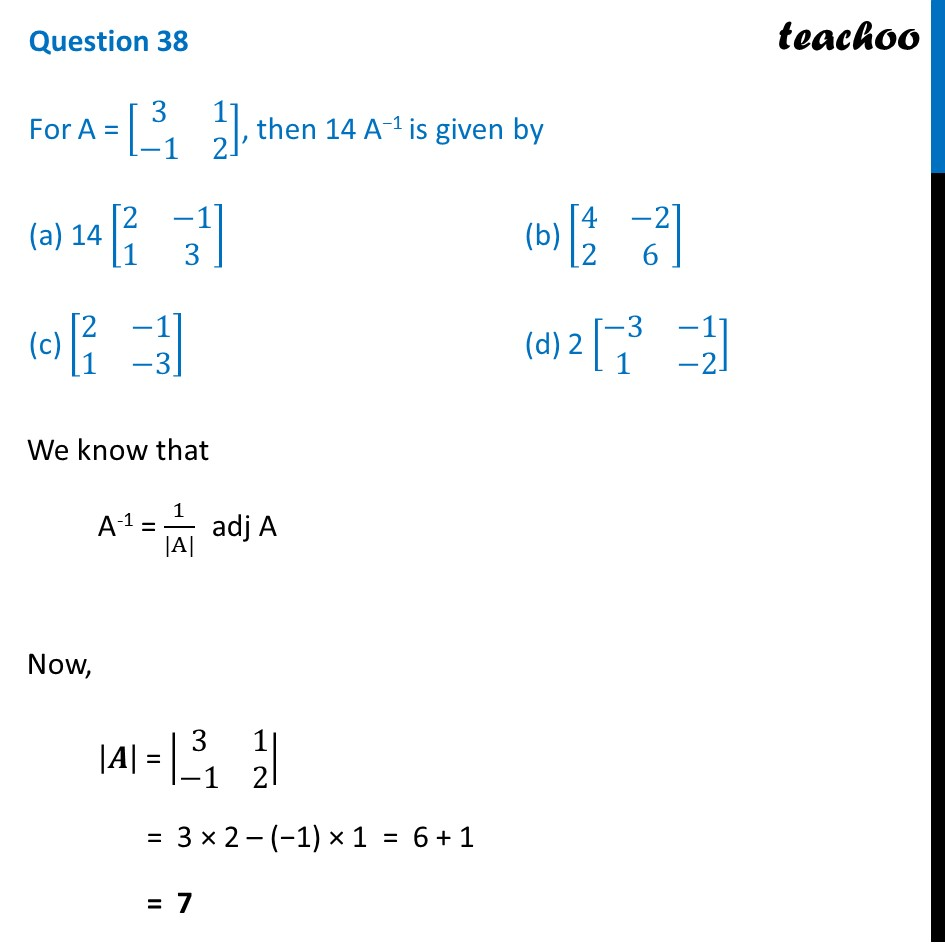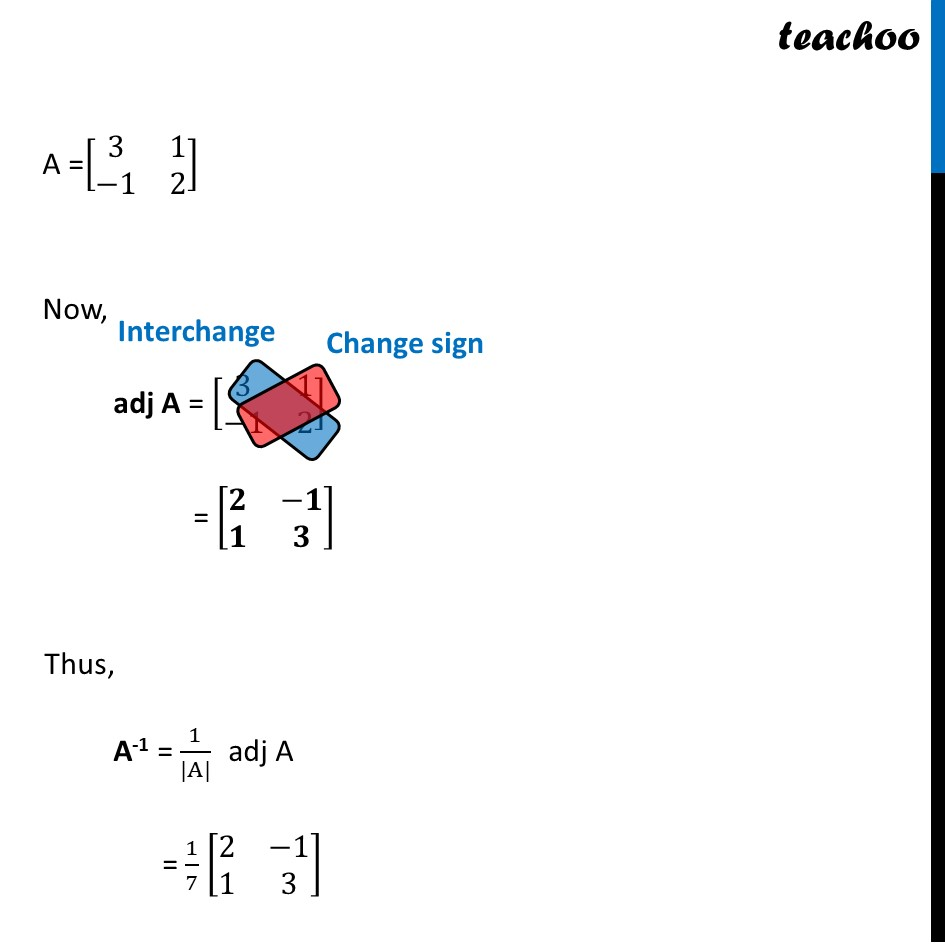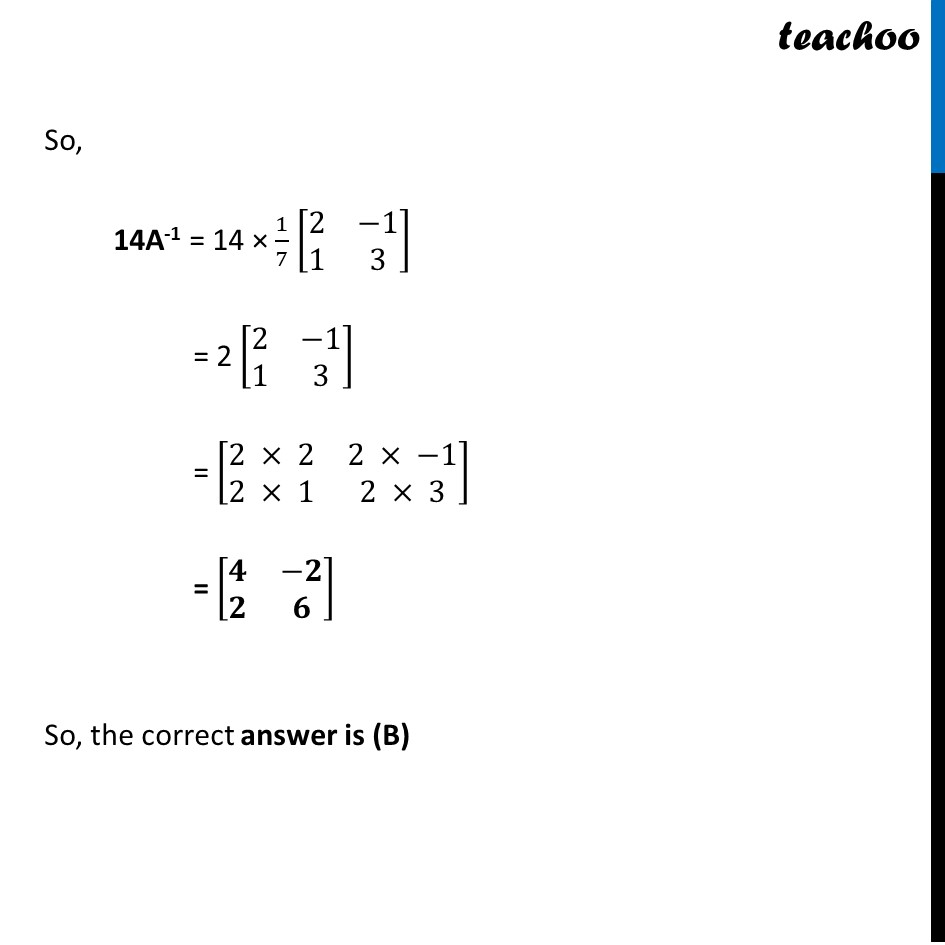CBSE Class 12 Sample Paper for 2022 Boards (MCQ Based - for Term 1)

Class 12
Solutions of Sample Papers and Past Year Papers - for Class 12 Boards

## (c) [8(2 -1 1 -3)]        (d) 2 [8(-3 -1 1 -2)]Learn in your speed, with individual attention - Teachoo Maths 1-on-1 Class

### Transcript

Question 38 For A = [■8(3&1@−1&2)], then 14 A−1 is given by (a) 14 [■8(2&−1@1&3)] (b) [■8(4&−2@2&6)] (c) [■8(2&−1@1&−3)] (d) 2 [■8(−3&−1@1&−2)] We know that A-1 = 1/(|A|) adj A Now, |𝑨| = |■8(3&1@−1&2)| = 3 × 2 – (−1) × 1 = 6 + 1 = 7 So, the correct answer is (B) A =[■8(3&1@−1&2)] Now, adj A = [■8(3&1@−1&2)] = [■8(𝟐&−𝟏@𝟏&𝟑)] Thus, A-1 = 1/(|A|) adj A = 1/7 [■8(2&−1@1&3)] So, 14A-1 = 14 × 1/7 [■8(2&−1@1&3)] = 2 [■8(2&−1@1&3)] = [■8(2 × 2&2 × −1@2 × 1&2 × 3)] = [■8(𝟒&−𝟐@𝟐&𝟔)] So, the correct answer is (B)